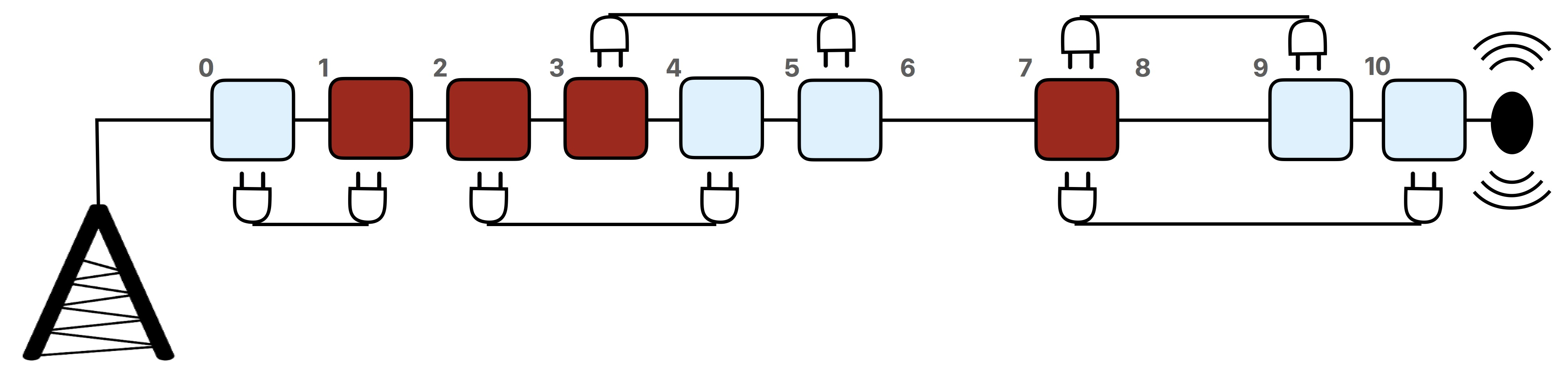# #321. 【IOI2017】Wiring

Maryam 是一位电机工程师。她正在为一座通讯塔设计接线方案。在这个塔上有一些分布在不同高度的连接点。一条电线可以用来将任何两个连接点接起来。每一个连接点都可以接上任意数目的电线。而连接点共有两种：分别为红色连接点及蓝色连接点。

1. 每个连接点上最少有一条电线连接到一个不同颜色的连接点上
2. 所用的电线的总长度为最短。

### 实现细节

 long long min_total_length(std::vector<int> r, std::vector<int> b)
• $r$: 一个长度为 $n$ 的数组，其内以升序排列着所有红色连接点的位置。
• $b$: 一个长度为 $m$ 的数组，其内以升序排列着所有蓝色连接点的位置。
• 这个子程序需返回在所有可能的连接方案中，最短电线总长度的那个方案的电线总长度作为其返回值。
• 请注意这个子程序的返回值的类型为 long long

### 例子• 图中以水平的方式表示出相关的通讯塔。
• 因题目打印是黑白色的，所以红色接点以较深色来表示，而蓝色接点以较浅色来表示。
• 图中有 $4$ 个红色的连接点，其位置分别为 $1,2,3$ 及 $7$。
• 图中有 $5$ 个蓝色的连接点，其位置分别为 $0,4,5,9$ 及 $10$。
• 该例的最优解的电线总长度为 $1+2+2+2+3=10$，所以子程序的返回值为 $10$。
• 注意共有两条电线连接在位置为 $7$ 的连接点上。

### 数据范围

• $1\leq n,m\leq 100\ 000$
• $0\leq r[i]\leq 10^9$（对于所有 $0 \leq i \leq n-1$）
• $0\leq b[i]\leq 10^9$（对于所有 $0 \leq i \leq m-1$）
• 数组 $r$ 及 $b$ 都已经按升序排好序。
• 在数组 $r$ 及 $b$ 的所有 $n+m$ 个值均是不同的。

$1$ $n,m\leq 200$ $7$
$2$ 所有红色接点的位置坐标小于任何蓝色接点的位置坐标 $13$
$3$ 在每 $7$ 个连续（接续）的连接点内必有最少一个红色接点及一个蓝色接点 $10$
$4$ 所有接点在 $[1,n+m]$ 范围内有不同的位置坐标 $25$
$5$ 无附加限制 $45$

### 评分程序样例

• 第 $1$ 行：$n,m$
• 第 $2$ 行：$r\ r\ ... \ r[n-1]$
• 第 $3$ 行：$b\ b\ ... \ b[m-1]$